# 4 3 Chemical Reactions Chemical Reactions Chemical reactions

• Slides: 8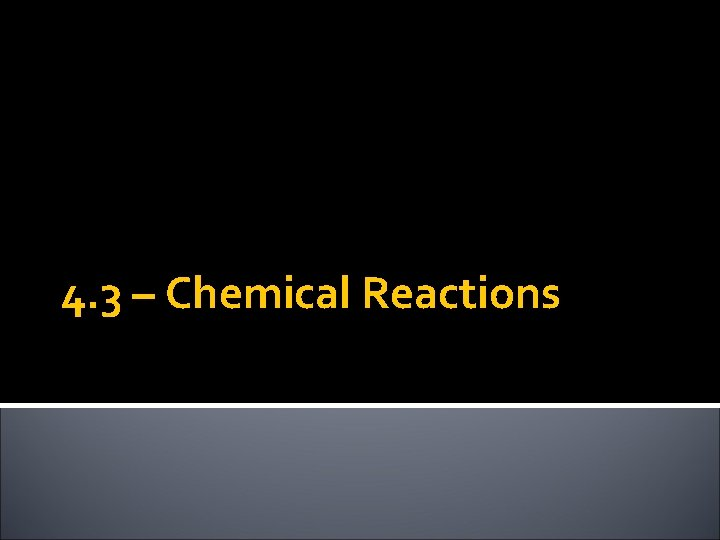4. 3 – Chemical Reactions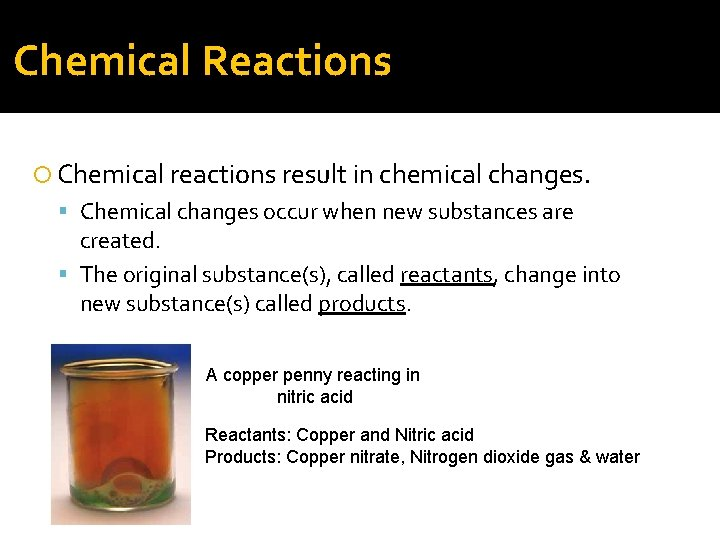Chemical Reactions Chemical reactions result in chemical changes. Chemical changes occur when new substances are created. The original substance(s), called reactants, change into new substance(s) called products. A copper penny reacting in nitric acid Reactants: Copper and Nitric acid Products: Copper nitrate, Nitrogen dioxide gas & water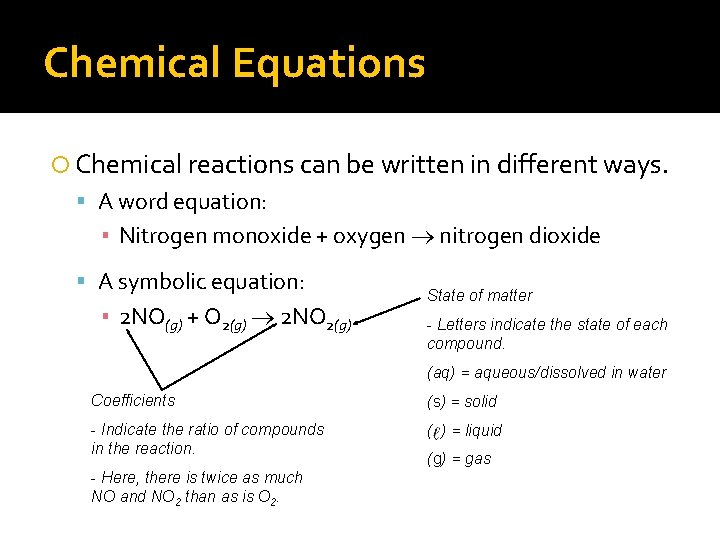Chemical Equations Chemical reactions can be written in different ways. A word equation: ▪ Nitrogen monoxide + oxygen nitrogen dioxide A symbolic equation: ▪ 2 NO(g) + O 2(g) 2 NO 2(g) State of matter - Letters indicate the state of each compound. (aq) = aqueous/dissolved in water Coefficients (s) = solid - Indicate the ratio of compounds in the reaction. ( ) = liquid - Here, there is twice as much NO and NO 2 than as is O 2. (g) = gas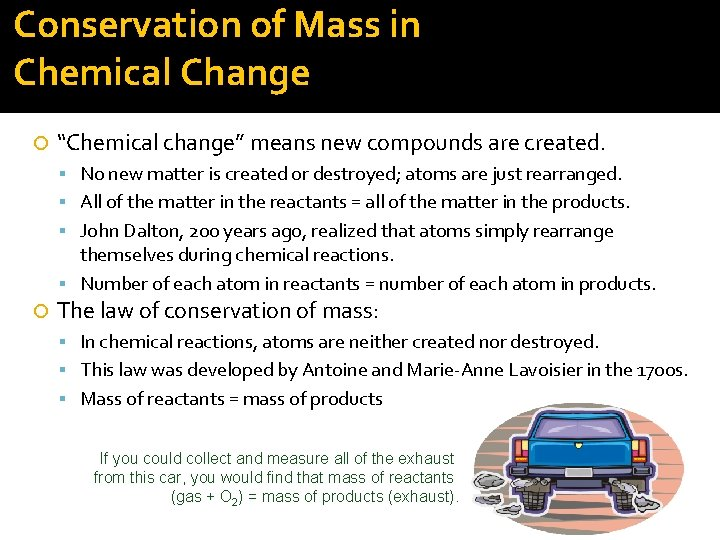Conservation of Mass in Chemical Change “Chemical change” means new compounds are created. No new matter is created or destroyed; atoms are just rearranged. All of the matter in the reactants = all of the matter in the products. John Dalton, 200 years ago, realized that atoms simply rearrange themselves during chemical reactions. Number of each atom in reactants = number of each atom in products. The law of conservation of mass: In chemical reactions, atoms are neither created nor destroyed. This law was developed by Antoine and Marie-Anne Lavoisier in the 1700 s. Mass of reactants = mass of products If you could collect and measure all of the exhaust from this car, you would find that mass of reactants (gas + O 2) = mass of products (exhaust).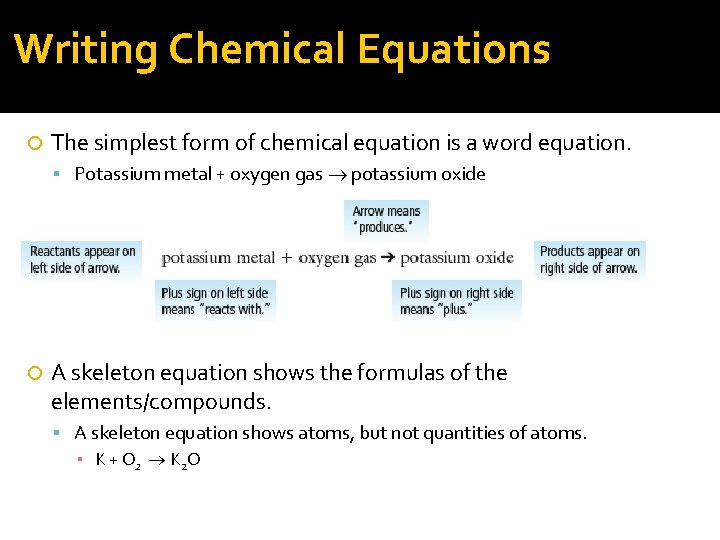Writing Chemical Equations The simplest form of chemical equation is a word equation. Potassium metal + oxygen gas potassium oxide A skeleton equation shows the formulas of the elements/compounds. A skeleton equation shows atoms, but not quantities of atoms. ▪ K + O 2 K 2 OBalancing Chemical Equations A balanced chemical equation shows all atoms and their quantities Balancing ensures that the number of each atom is the same on both sides of the reaction arrow. Always use the smallest whole-number ratio. ▪ Skeleton equation: ▪ K + O 2 K 2 O reactants: 1 K and 2 O Not balanced! products: 2 K and 1 O ▪ Balanced equation: ▪ 4 K + O 2 2 K 2 O reactants: 4 K and 2 O Balanced! The amount of each atom is the same on products: 4 K and 2 O both sides!Exploding Balloons. . . We will be looking the following reaction: Word equation: Zinc metal + hydrochloric acid zinc chloride + hydrogen gas then Hydrogen gas + oxygen gas waterQuestions: What were the reactants in each reaction? What were the products in each reaction? The word equations for the reactions were: Zinc metal + hydrochloric acid zinc chloride + hydrogen gas Hydrogen gas + oxygen gas water The skeleton equations were: Zn(s) + HCl(aq) Zn. Cl₂(aq) + H₂(g) H 2(g)+ O 2(g) H 2 O( ) How many Zn, H and Cl atoms are on each side of the equation?